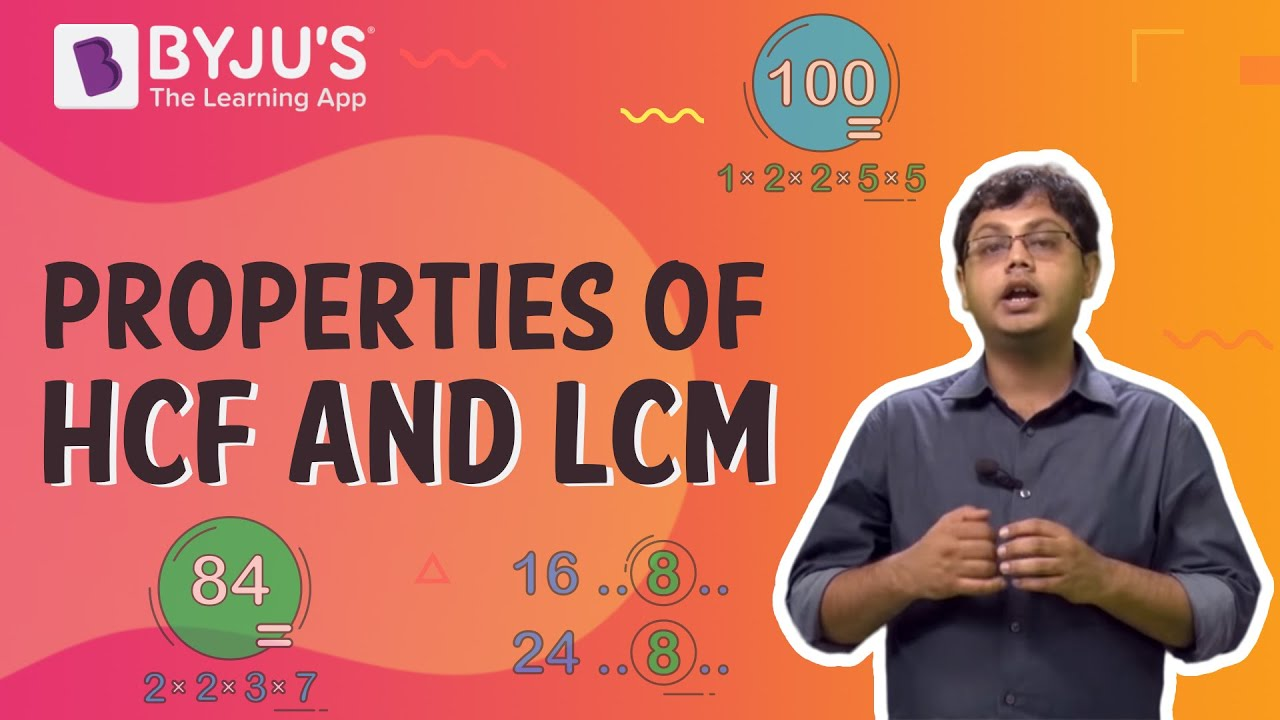# The LCM of two numbers is 2516 and the square root of their HCF is 2, find the product of the two numbers.

The largest common divisor or Highest Common Factor (HCF) of two or more positive integers, as the mathematics rules dictate, appears to be the largest positive integer that divides the numbers without leaving a remainder.

In arithmetic, LCM (a,b) is the least common multiple of two numbers, a and b. And the LCM is the smallest or least positive integer that is divisible by both a and b.

Given:

• LCM of two numbers is 2516
• Square root of their HCF is 2

By the formula of LCM for two numbers we have:

LCM (a, b) = (a × b)/HCF (a, b)

The HCF of the two numbers = 2 × 2 = 4

(a × b) = LCM (a, b) × HCF (a, b)

(a × b)  = 2516 × 4

(a × b) = 1064

Therefore, the product of the two numbers is 1064.

∴ The product of the two numbers, whose LCM is 2516 and the square root of their HCF is 2 is 1064.

Check out the video given below to know more about the properties of  HCF and LCM### Articles to Explore:(1)(0)